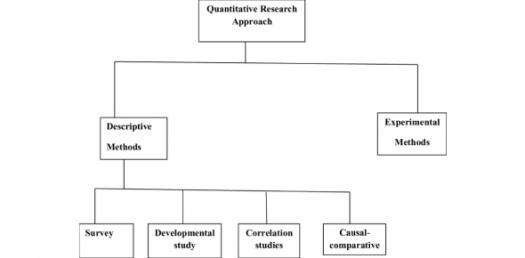# Quantitative Research Methods

20 Questions | Total Attempts: 1280SettingsQuantitative Research Methods + UOPX RES750 material

• 1.
A true, absolute zero exists for which level of measurement?
• A.

Nominal

• B.

Ordinal

• C.

Interval

• D.

Ratio

• 2.
You spent 30% of your HR budget on benefits three years ago, 35% two years ago, and 40% last year. When was the most money spent on benefits?
• A.

Three years ago

• B.

Two years ago

• C.

Last year

• D.

It cannot be determined from the information provided

• 3.
You have been given the task of examining the effect of a pay-for-performance project on employee productivity. You have obtained a “productivity rating score” for all program participants. Which of the following best describes your data?
• A.

The data represent a sample.

• B.

The data represent a population.

• C.

Productivity rating scores only produce nominal data.

• D.

Productivity rating scores always produce ordinaal data.

• 4.
What is the mode for the following set of numbers? 15, 18, 13, 19, 25, 24, 26, 14, 25, 35, 12, 99, 54, 13, 22, 19 and 13
• A.

19

• B.

26

• C.

24

• D.

13

• 5.
A range can best be described as which of the following?
• A.

The central tendency of values between data points.

• B.

The difference between the maximum and minimum value in a data set.

• C.

A tool used to measure the difference in a data set based on the middle 50%.

• D.

The only measure of variability appropriate for ratio data.

• 6.
What type of regression model does the following equation suggest? y = a + b1x1
• A.

Multiple factor regression.

• B.

Nonlinear regression.

• C.

Bivariate regression.

• D.

Curvilinear regression.

• 7.
How does quantitative research facilitate qualitative research?
• A.

Quantitative research identifies the groups of people that must be interviewed.

• B.

Quantitative research increases the chances that people will take part in an interview.

• C.

Quantitative research balances positivist and post-positivist research approaches.

• D.

Quantitative research balances positivist and post-positivist research approaches.

• 8.
Which of the following correlations indicates the weakest relationship?
• A.

+.10

• B.

+.83

• C.

-.74

• D.

-.21

• 9.
A statement that predicts a cause-and-effect relationship between variables is known as the _____________.
• A.

Null hypothesis.

• B.

Experimental hypothesis.

• C.

Independent variable.

• D.

Dependent variable.

• 10.
What is the mean of the following numbers: 2, 7, 6, 5, 4, 2, 8, 9 ,1, 6
• A.

2

• B.

4

• C.

5

• D.

7

• 11.
Correlation coefficient provides all of the following information, except :
• A.

Whether or not there is a relationship between the variables.

• B.

The strength of the relationship between the variables.

• C.

The cause of the relationship between the variables.

• D.

The direction of the relationship between the variables.

• 12.
Another name for a bar graph is:
• A.

Polygon

• B.

Histogram

• C.

Normal curve

• D.

Line graph

• 13.
A table that shows how often different scores appear in a set of scores is called a frequency:
• A.

Polygon

• B.

Histogram

• C.

Normal curve

• D.

Distribution

• 14.
All the following are components required for estimating power (1-beta) of a study, EXCEPT
• A.

The population effect size

• B.

Sample size

• C.

Level of significance (alpha)

• D.

Standard deviation

• 15.
When doing power analysis in ANOVA context, the estimate of effect size is
• A.

Eta-squared

• B.

Correlation coefficient

• C.

Standard Error

• D.

Chi-squire value

• 16.
Analysis of Variance (ANOVA) test is used to
• A.

Test the difference between two independent group means

• B.

Test the difference between two related group means

• C.

Test the difference among the means of 3+ independent groups

• D.

Test the difference in ranks of scores of 3+ independent groups

• 17.
Effect size is used to calculate:
• A.

Reliability

• B.

Sample size

• C.

Internal consistency

• D.

Predictive validity

• 18.
The F-statistic is calculated in:
• A.

ANOVA

• B.

T-test

• C.

Correlation analysis

• D.

Chi-squire test

• 19.
Which of the following is a nonparametric "Analysis of Variance"?
• A.

Mann-Whitney U test

• B.

Wilcoxon Rank test

• C.

Kruskal-Wallis test

• D.

Friedman's test

• 20.
Chi-square test is used to test:
• A.

Difference in proportions

• B.

Difference in means of two independent variables

• C.

Relationship between two bivariate variables

• D.

Difference in means of three or more set of variables

Related TopicsBack to top